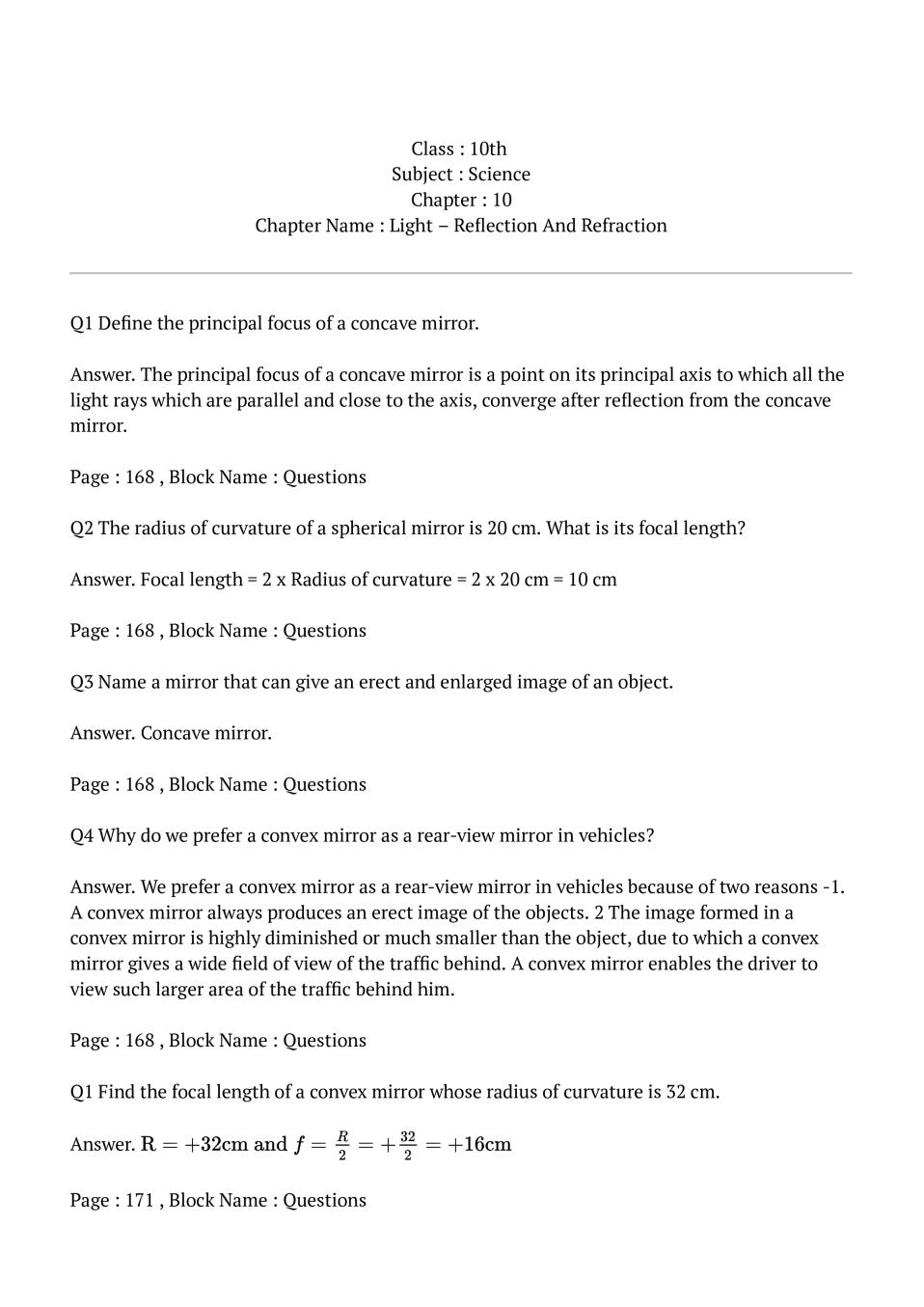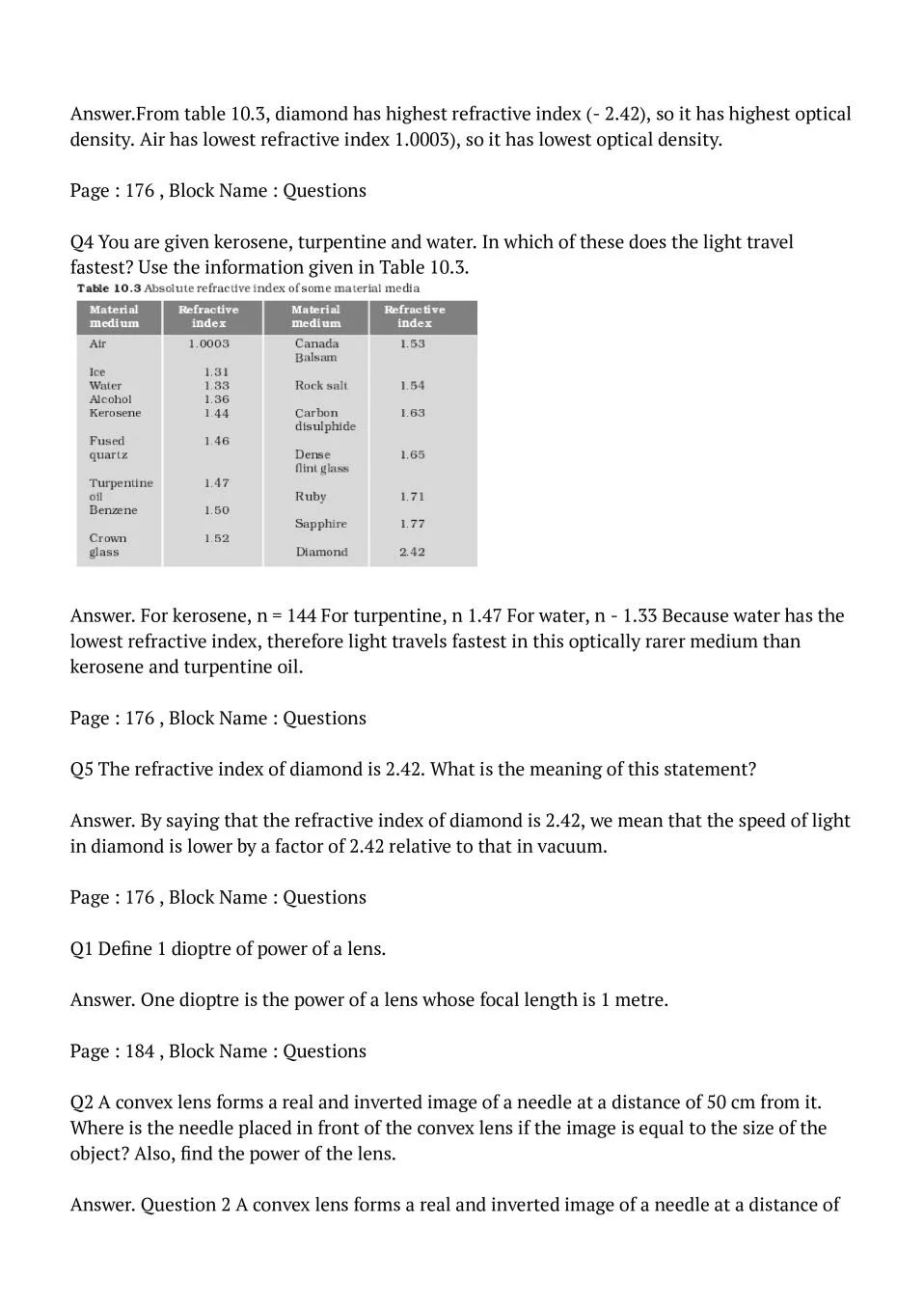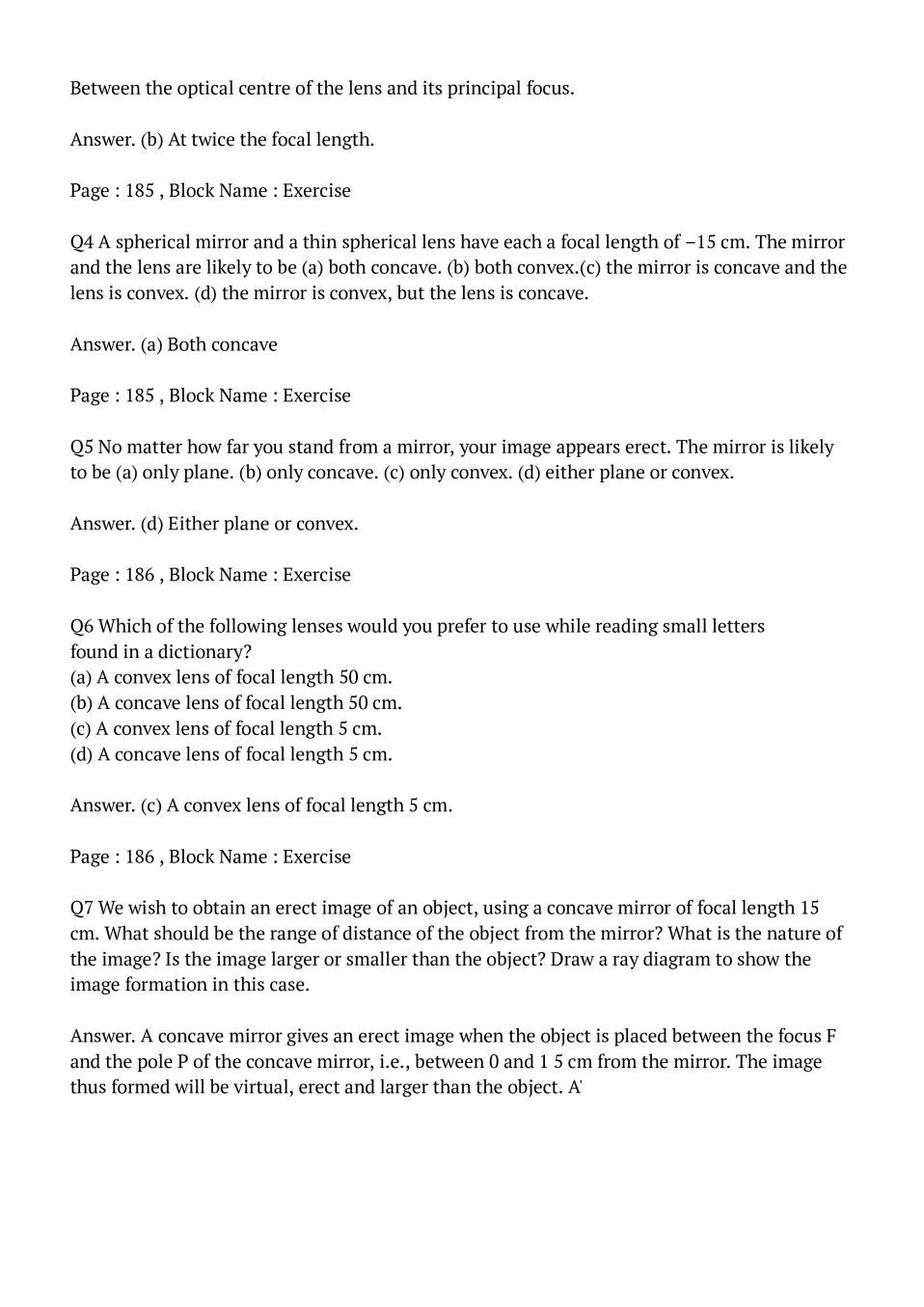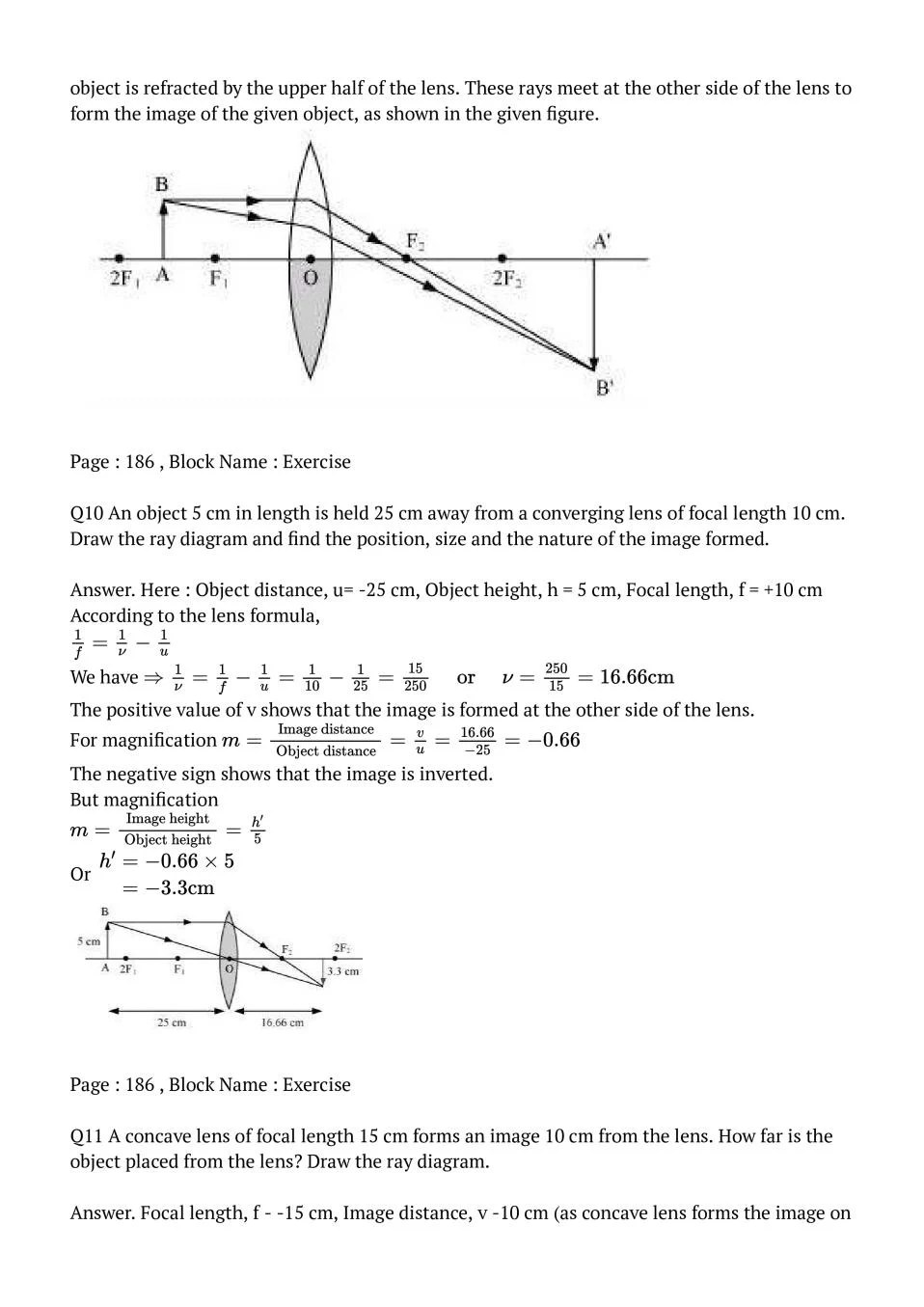# NCERT Solutions Class 10 Science Chapter 10 Light – Reflection and Refraction

Here you can get free NCERT Solutions for Class 10 Science, of Chapter 10 Light – Reflection and Refraction. All NCERT Book Solutions are given here exercise wise for Light – Reflection and Refraction. NCERT Solutions are helpful in the preparation of several school level, graduate and undergraduate level competitive exams. Practicing questions from NCERT Science Book, its Solutions for Class 10 Chapter 10 Light – Reflection and Refraction is proven to enhance your subject skills.

 Class: 10th Class Chapter: Chapter 10 Name: Light – Reflection and Refraction Subject: Science

## NCERT Solutions Class 10 Science Chapter 10 – Light – Reflection and Refraction

NCERT Class 10 Solutions Chapter 10 – Light – Reflection and Refraction are given below.

NCERT Solutions for Class 10 Science Chapter 10 Light – Reflection and Refraction### Class 10 Science Chapter 10 NCERT Solutions

Get step by step solutions for the questions given in class 10 Science textbook from the latest NCERT as per board exam guidelines.

### Class 10 Science Chapter 10 Important Topics

1. Reflection of Light
2. Spherical Mirrors
–  Image Formation by Spherical Mirrors
–  Representation of Images Formed by Spherical Mirrors Using Ray Diagrams
–  Sign Convention for Reflection by Spherical Mirrors
–  Mirror Formula and Magnification
3. Refraction of Light
–  Refraction through a Rectangular Glass Slab
–  The Refractive Index
–  Refraction by Spherical Lenses
–  Image Formation by Lenses
–  Image Formation in Lenses Using Ray Diagrams
–  Sign Convention for Spherical Lenses
–  Lens Formula and Magnification
–  Power of a Lens

### Summary Chapter 10 Class 10 Science

• Light seems to travel in straight lines.
• Mirrors and lenses form images of objects. Images can be either real or virtual, depending on the position of the object.
• The reflecting surfaces, of all types, obey the laws of reflection. The refracting surfaces obey the laws of refraction.
• New Cartesian Sign Conventions are followed for spherical mirrors and lenses.
• Mirror formula, (1/v)+(1/u)=1/f, gives the relationship between the object-distance (u), image-distance (v), and focal length (f) of a spherical mirror.
• The focal length of a spherical mirror is equal to half its radius of curvature.
• The magnification produced by a spherical mirror is the ratio of the height of the image to the height of the object.
• A light ray travelling obliquely from a denser medium to a rarer medium bends away from the normal. A light ray bends towards the normal when it travels obliquely from a rarer to a denser medium.
• Light travels in vacuum with an enormous speed of 3×10^8 m/s. The speed of light is different in different media.
• The refractive index of a transparent medium is the ratio of the speed of light in vacuum to that in the medium.
• In case of a rectangular glass slab, the refraction takes place at both air-glass interface and glass-air interface. The emergent ray is parallel to the direction of incident ray.
• Lens formula, (1/v)+(1/u)=1/f, gives the relationship between the object-distance (u), image-distance (v), and the focal length (f) of a spherical lens.
• Power of a lens is the reciprocal of its focal length. The SI unit of power of a lens is dioptre.

Exercise Covered under Science Book Class 10 Science Chapter 10 NCERT Solutions

Here on this page we have covered following exercises from NCERT Science Book for Class 10 Science Chapter 10 Light – Reflection and Refraction

 Questions All Questions Solved Exercises 17 Questions with Solutions

We also suggest you to practice all the solved examples given in the NCERT book to clear your concepts on Light – Reflection and Refraction. You can buy NCERT Books for Class 10 Science online for doorstep delivery.

### NCERT Solutions Class 10 Chapter Wise

If you want NCERT solutions of any topic other than Chapter 10 Light – Reflection and Refraction, check it from here. Science Book by NCERT has all chapters that are in your Class 10 syllabus.

### NCERT Solutions Class 10 All Subjects

All NCERT Solutions for Subjects other than Science are provided below:

 English Hindi Science Maths Social Science

### Other Classes NCERT Solutions

If you want NCERT solutions of any class other than Class 10, check it from here.